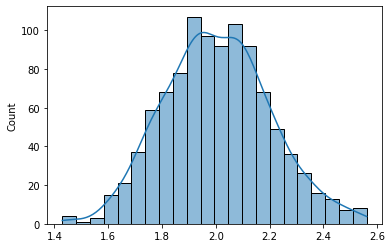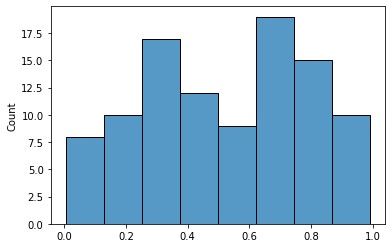# Working with Random Numbers in Numpy

## Working with Random Numbers in NumpyRandom is a module in the Numpy library for providing random numerical data in any required data structure. It contains simple functions/methods to generate random numbers, permutations and probability distributions. In this tutorial, we will understand how to use these functions and create random data as per our needs.

These features are based on PRNG(Pseudo Random Number Generation) Algorithms. To suffice, the PRN generator uses mathematical formulas to produce sequence of random numbers using an arbitrary seed state. This helps to reproduce the same numbers at one's convenience.

Let's begin by importing numpy.

import numpy as np

### Simple Random Functions

Let's go over these functions one by one:

#### numpy.random.rand()

• This function allows random numbers generation in a desired shape (Provided in the argument).
• Syntax: numpy.random.rand($$d_1, d_2,..d_n$$).
• This will typically return values from a uniform distribution over 0,1.

Uniform Distribution: When the probability of each value is equally likely, example, rolling a fair die.• If no argument is provided, the function will return a single float value.
a = np.random.rand()
b = np.random.rand(2)
print(a, ",", b)
0.3224876833345295 , [0.80038351 0.02511512] 
#creating an array of dimensions (4[2,2])
a = np.random.rand(4,2,2)
a
array([[[0.5709071 , 0.23116103],
[0.17869279, 0.03264607]],

[[0.38270662, 0.99443131],
[0.60835673, 0.16401943]],

[[0.30376811, 0.7839127 ],
[0.51879298, 0.98232092]],

[[0.68055995, 0.36071315],
[0.793239  , 0.20790045]]])

#### numpy.random.randn()

• randn() is simillar to rand() and provides an array of the mentioned dimensions except that, this function returns random floats sampled from a Standard Normal Distribution.• The Standard Normal Distribution is the Gaussian(Normal) Distribution with mean 0 and variance 1.

• In any case the argument provided are float, they are first converted to int.

a = np.random.randn()
b = np.random.randn(2,2)
print("a = ", a, ",\n"," b = ", b)
a = -0.2597162279610976 , b = [[ 0.26918118 -0.72787076] [ 0.11213333 1.33860462]] 

#### numpy.random.randint()

• This function takes in a low and a high argument & returns random integers from the half-open interval. i.e [low, high) $$\equiv$$ low(inclusive), high (exclusive)
• You can mention size of required array in the size argument.
a = np.random.randint(3)
b = np.random.randint(low=2,high=7, size=3)
print("a = ", a, ",\n"," b = ", b)
a = 1 , b = [4 4 2] 
• If high is none, then the result will range within [0, low).
a = np.random.randint(1, size=8)
a
array([0, 0, 0, 0, 0, 0, 0, 0])

#### numpy.random.random() & random_sample()

• Above mentioned both functions have the same functionality and can be used inter-changeably.
• These functions return random floats in the half open interval's continous uniform distribution of [0.0, 1.0).
• You can specify the size (optional) argument to return an array of a specific size.
a = np.random.random()
a
0.4502639286171949
b = np.random.random_sample(size=8)
b
array([0.78564775, 0.12364401, 0.09253097, 0.20851597, 0.66741015,
0.19139018, 0.03421576, 0.51810702])

Note: Along with these two, numpy.random.sample() and numpy.random.ranf() and return the same results.

#### numpy.random.choice()

• The choice function generates a random sample from a given 1-D array in a argument.

• You can use the size argument to mention the output shape.

• If for the input, an array is provided the values in the output come from this input array. But if an integer is provided for the input, random values are generated from numpy.arange(input_int).

(You can check out the functionality of np.arange() here: https://numpy.org/doc/stable/reference/generated/numpy.arange.html?highlight=arange#numpy-arange)

a = np.random.choice(15,4)
a 
array([1, 3, 5, 1])
b = np.random.choice(a, 2)
b 
array([1, 3])
• The choice function also provides the replace:boolean argument, which is True by default. Which means that a value of input array a, can selected multiple times.

• The p argument ≡ probabilities associated with each entry in a. i.e, You can provide another argument stating the probability for selecting each item in a.

Note: Make sure that the probabilites you provide for each entry in a have the same size as a and sum upto 1.

a = np.random.choice(7, 4)
b = np.random.choice(a, 2, replace=False, p = [0.1, 0.1, 0.5, 0.3])
b

array([6, 6])

#### numpy.random.bytes()

• This function simply returns random bytes of the size mentioned in the argument.

• random.bytes(length)

• This can especially be used to generate API keys and passwords.

a = np.random.bytes(5)
b = np.random.bytes(50)

print("a = ", a, ",\n"," b = ", b)
a = b'\xde\x87\xa2\xfb)' , b = b'\x10\x01>-\xae\x08WY\xfc\x00\x99\x96\x83Q)&\xbf3f\xf1I\xe8\x99\xd3\xf2t\xf5\xdb\x8e\r>\xc5\xaee\x80[CM\t4\xc9\x19L\xa6\xd2\xd33|\x8d\x99' 

### Random Distributions

Random provides a myriad of methods to access probability distributions. You can access the whole list of methods provided by random here: https://numpy.org/doc/1.16/reference/routines.random.html#distributions

Let's look at a few examples:

#### Normal Distribution

• numpy.random.normal(loc, scale, size)

• Like the name suggests, this function will return random samples from a normal (Gaussian) Distribution.

• loc: Mean, scale: Std Deviation, size: Output shape

m , sd = 2 ,0.2
n = np.random.normal(m, sd, 1000)

Using Seaborn to plot our distribution:

import matplotlib.pyplot as plt
import seaborn as sns

sns.histplot(n, kde=True);#### Binomial Distribution

• numpy.random.binomial(n,p,size)
• n: Number of Trials at a time
• p: Probability of Success

Let's create a distribution of number of heads in a coin tossed a 100 times with probability = 0.5.

b = np.random.binomial(n = 2, p = 0.5, size=100)
b 
array([1, 0, 0, 1, 1, 1, 0, 1, 1, 1, 1, 2, 1, 2, 0, 2, 0, 1, 1, 1, 1, 0,
2, 1, 1, 0, 1, 1, 2, 2, 1, 0, 1, 1, 0, 1, 1, 1, 1, 1, 1, 1, 1, 1,
2, 2, 1, 1, 2, 0, 1, 2, 1, 1, 0, 2, 1, 0, 1, 0, 1, 1, 0, 1, 1, 2,
1, 0, 1, 1, 2, 1, 2, 1, 0, 2, 2, 1, 2, 0, 0, 0, 1, 0, 2, 2, 1, 1,
0, 1, 0, 1, 1, 2, 2, 0, 1, 1, 0, 1])

Similarly, we can create data for a dice with each digit having a probability of 1/6, being tossed a 100 times.

b = np.random.binomial(n=6, p=1/6, size=100)
b
array([1, 0, 1, 1, 1, 2, 1, 1, 2, 3, 1, 1, 1, 0, 3, 2, 2, 3, 0, 1, 2, 1,
0, 0, 0, 2, 0, 1, 0, 3, 0, 1, 1, 1, 1, 0, 1, 0, 1, 1, 0, 2, 1, 1,
3, 2, 1, 0, 0, 1, 1, 1, 0, 1, 0, 0, 1, 2, 1, 0, 3, 1, 2, 2, 1, 2,
2, 0, 2, 0, 1, 1, 1, 0, 0, 2, 3, 2, 0, 1, 0, 3, 1, 0, 1, 2, 3, 2,
2, 1, 1, 1, 0, 0, 0, 1, 1, 1, 3, 0])

#### Uniform Distribution

• numpy.random.uniform(low, high, size)

• This function draws samples from a half open interval of the uniform distribution between the low and high argument. i.e [low, high).

• Hence, any value btw low and high, is equally likely to be chosen or

• By default low is 0 and high is 1.

u = np.random.uniform(size = 100)

sns.histplot(u);# Execute this to save new versions of the notebook
jovian.commit(project="numpy-random-module")
[jovian] Detected Colab notebook... [jovian] Uploading colab notebook to Jovian... Committed successfully! https://jovian.ai/himani007/numpy-random-module 
'https://jovian.ai/himani007/numpy-random-module'

### Random Generator

#### RandomState

• numpy.random.RandomState(seed)
• This specific function is a container which provides access to a wide variety of probability distributions using the Mersenne Twister PRNG.
• In simple words, RandomState uses the Mersenne Twister Pseudo Random Number Generator within just like Random but the only difference being, that RandomState provides a much larger number of probability distributions to choose from.

You can access the entire list of Methods provided by RandomState here: https://numpy.org/doc/1.16/reference/generated/numpy.random.RandomState.html#numpy-random-randomstate

#### Seed

• np.random.seed(seed)
• The random number generator needs a number to start with, which we fix using seed.
• This method is used when we want to reproduce the same set of numbers.
• We can initialize a seed to generate a random set of numbers, and with the same seed value, a simillar set of numbers will be generated everytime.
np.random.seed(10)
a = np.random.rand()
a
0.771320643266746
np.random.seed(10)
b = np.random.rand()

print(a,b)
0.771320643266746 0.771320643266746 

Typically a seed is used when we want to produce the same random numbers throughout the notebook. So after declaring the seed once in the beginning, you can expect different random number sequences accross the notebook, yet the same sequences everytime you re-run your notebook.

### PermutationsThe Random module provides the following functions for permutations:

#### Shuffle

• numpy.random.shuffle(x), where x: Array
• This function is used to shuffle the array provided in the argument.
arr = np.random.randint(8, size=5)
arr
array([0, 3, 2, 1, 4])
np.random.shuffle(arr)
arr
array([1, 2, 4, 3, 0])

Note: In case of a multidimensional array, the arrays are shuffled along the first axis.

#### Permutation

• numpy.random.permutation(x), where x: int or array.

• This function can be used as shuffle for rearranging or if x is an integer, then the function will first call np.arange(x), and then shuffle the array.

np.random.permutation(8)
array([7, 5, 4, 6, 2, 3, 0, 1])
a = np.random.permutation(8)
np.random.permutation(a)
array([4, 6, 7, 1, 2, 5, 0, 3])

### Conclusion

Random module is suitable when there is need for a huge number of random numbers especially when the same sequence is needed repeatedly. In this tutorial we learned examples on numpy.random's:

• rand()
• randn()
• randint()
• random(), sample()
• choice()
• bytes()

### References

jovian.commit(project="numpy-random-module")
[jovian] Detected Colab notebook... [jovian] Uploading colab notebook to Jovian... Committed successfully! https://jovian.ai/himani007/numpy-random-module 
'https://jovian.ai/himani007/numpy-random-module'Himania year ago